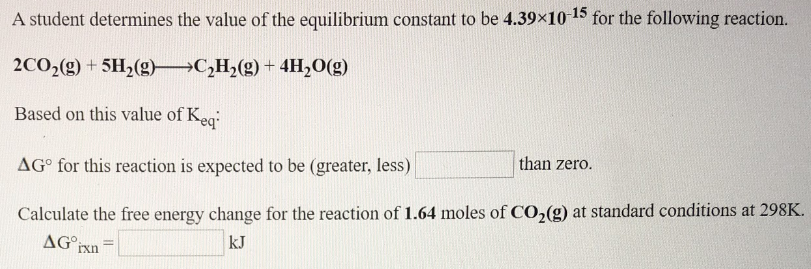# A student determines the value of the equilibrium constant to be 4.39x10^-15 for the following reaction. 2CO2(g) + 5H2(g) → C2H29g) + 4H2O(g) Based on this value of Keq: ΔG° for this reaction is expected to be (greater, less) ____ than zero. Calculate the free energy change for the reaction of 1.64 moles of CO2(g) at standard conditions at 298K. ΔG°rxn = ____ kJ.